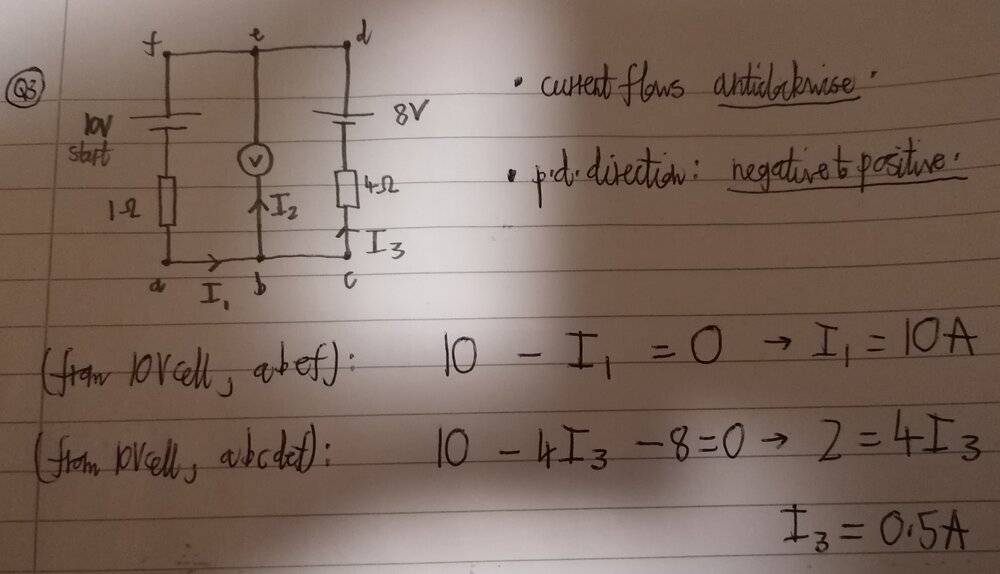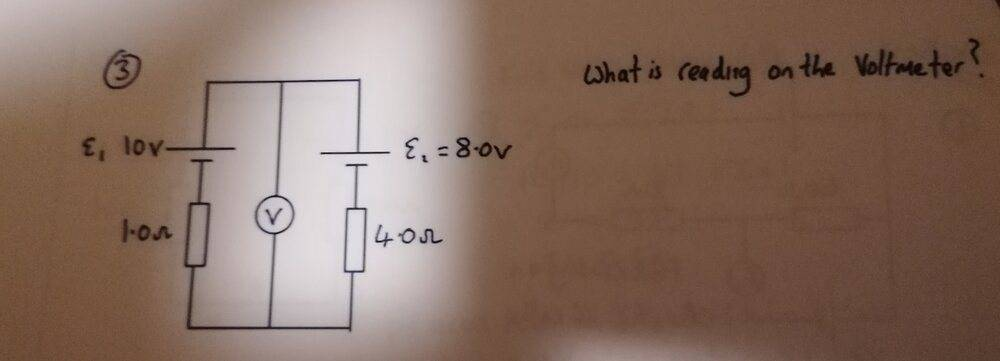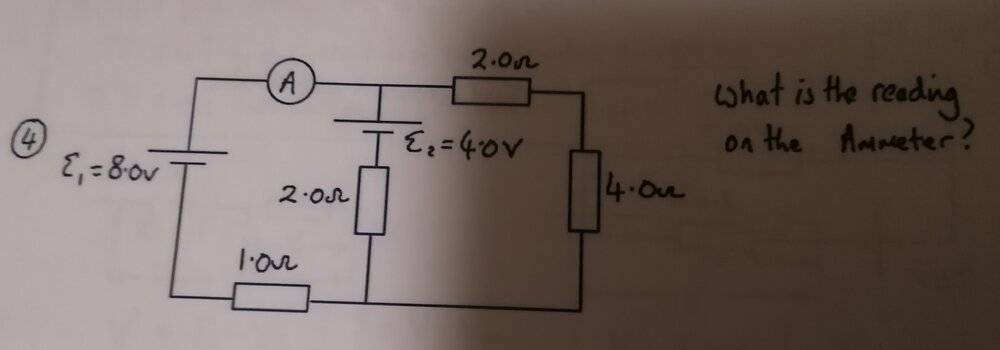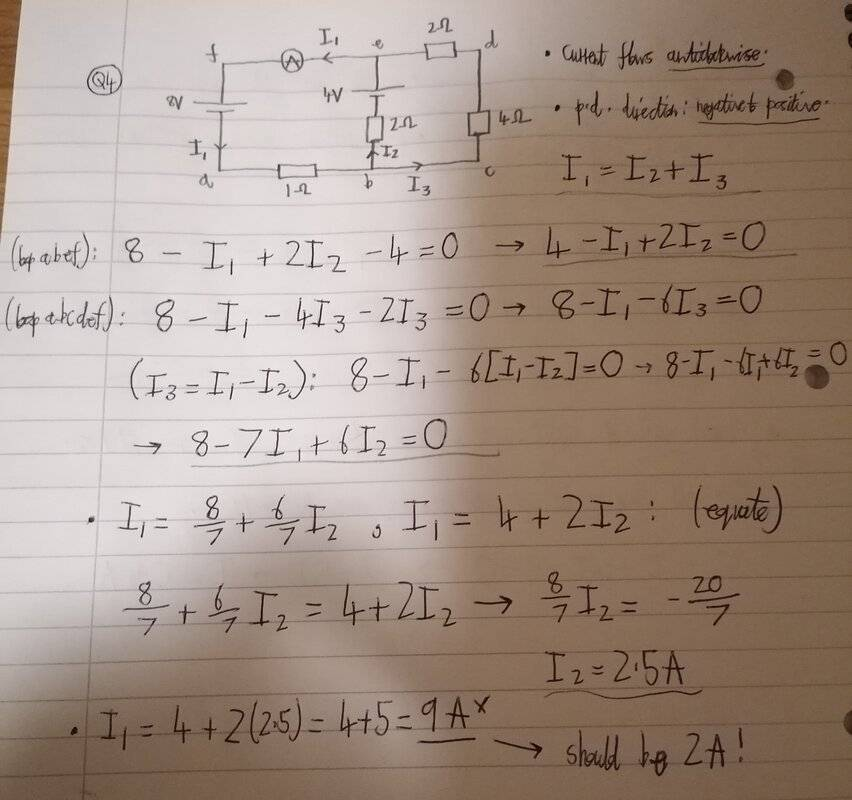# Need help with a Kirchhoff's Laws circuit problem

TomK
Homework Statement:
Can't do circuit problems with 2+ cells consistently. It works for some questions and doesn't for others. I'm guessing this is because my mistakes in one question end up cancelling each other out. I don't get it.
Relevant Equations:
https://www.ocr.org.uk/Images/536433-data-sheet.pdf
I wasn't taught how to do it at A-Level in-detail, because there are no questions that expect you to use them in a circuit problem. They only need you to know what the first and second laws are in words (i.e. conservation of charge and energy).

I tried looking it up on YT, but it's confusing. All I know is this:

1) Choose a direction for current to flow (CW or ACW) - consistent for every loop.

2) Choose a p.d. direction (+ to -, or - to +) - consistent for every loop.

3) current into a junction = current out of same junction.

4) sum of potentials in a closed loop = 0. This doesn't make sense to me, but I use it anyway.

5) If p.d. and current direction are against each other, add emf and component potentials. If p.d. and current direction are in same direction, subtract component potentials from emf.

I don't know what counts as a loop. Does it have to start and end at the same cell, or can a loop not involve a cell at all?

Whenever I have a loop that has a second cell in the way, I check if its p.d. direction opposes that of my starting cell. If it does, I subtract its potential from the emf of the starting cell.

I still can't do this question, though.The answer is 9.6V, but I can't see why.

Homework Helper
Gold Member
2022 Award
Homework Statement:: Can't do circuit problems with 2+ cells consistently. It works for some questions and doesn't for others. I'm guessing this is because my mistakes in one question end up cancelling each other out. I don't get it.
Relevant Equations:: https://www.ocr.org.uk/Images/536433-data-sheet.pdf

I wasn't taught how to do it at A-Level in-detail, because there are no questions that expect you to use them in a circuit problem. They only need you to know what the first and second laws are in words (i.e. conservation of charge and energy).

I tried looking it up on YT, but it's confusing. All I know is this:

1) Choose a direction for current to flow (CW or ACW) - consistent for every loop.

2) Choose a p.d. direction (+ to -, or - to +) - consistent for every loop.

3) current into a junction = current out of same junction.

4) sum of potentials in a closed loop = 0. This doesn't make sense to me, but I use it anyway.

5) If p.d. and current direction are against each other, add emf and component potentials. If p.d. and current direction are in same direction, subtract component potentials from emf.

I don't know what counts as a loop. Does it have to start and end at the same cell, or can a loop not involve a cell at all?

Whenever I have a loop that has a second cell in the way, I check if its p.d. direction opposes that of my starting cell. If it does, I subtract its potential from the emf of the starting cell.

I still can't do this question, though.

View attachment 270531View attachment 270532

The answer is 9.6V, but I can't see why.
You cannot assume there is a 10V drop across the 1Ω resistor. That's a voltmeter in the middle, so cannot be counted as completing a circuit, i.e. I2 is zero.
You can take that link out, leaving you with two resistors in series and two (opposing) emfs in series.

TomK
You cannot assume there is a 10V drop across the 1Ω resistor. That's a voltmeter in the middle, so cannot be counted as completing a circuit, i.e. I2 is zero.
You can take that link out, leaving you with two resistors in series and two (opposing) emfs in series.

I thought that too, since we should assume the voltmeter is of infinite resistance. This is what I have written:

10 - I - 4I - 8 = 0
5I = 2
I = 0.4A

How do I calculate the potential difference now?

Homework Helper
Gold Member
2022 Award
I thought that too, since we should assume the voltmeter is of infinite resistance. This is what I have written:

10 - I - 4I - 8 = 0
5I = 2
I = 0.4A

How do I calculate the potential difference now?
Calculate the voltage drop across one resistor.

TomK
Calculate the voltage drop across one resistor.

The calculation works out now.

I wanted to ask: why does the p.d. on the 'left side' (with the 10V cell) have to be the same as the p.d. on the 'right side' (with the 8V cell)? I know the voltmeter is in-parallel with both sides. It's confusing for me to think about, because the voltmeter is sandwiched between what would be a series circuit.

In a circuit problem, will you ever have to write an equation for a loop with no cell? I asked before: does a loop have to start and end at a cell?

I was doing this other question involving Kirchhoff's Laws, but I must have made a mistake somewhere. Would you be able to help? The answer should be 2A.Homework Helper
Gold Member
2022 Award
why does the p.d. on the 'left side' (with the 10V cell) have to be the same as the p.d. on the 'right side'
Consider the four corners of the bounding rectangle. Voltage top left=voltage top right; voltage bottom left =voltage bottom right.
does a loop have to start and end at a cell?
Yes. It has to be a circuit, and it has to include something that will create a potential difference even when there is no current.
How come it is -I1 and -4I3 but +2I2 ?

TomK
Consider the four corners of the bounding rectangle. Voltage top left=voltage top right; voltage bottom left =voltage bottom right.

Yes. It has to be a circuit, and it has to include something that will create a potential difference even when there is no current.

How come it is -I1 and -4I3 but +2I2 ?